Courses

# Test: Fluctuating Load Consideration For Design & Miscellaneous - 1

## 20 Questions MCQ Test RRB JE for Mechanical Engineering | Test: Fluctuating Load Consideration For Design & Miscellaneous - 1

Description
This mock test of Test: Fluctuating Load Consideration For Design & Miscellaneous - 1 for Mechanical Engineering helps you for every Mechanical Engineering entrance exam. This contains 20 Multiple Choice Questions for Mechanical Engineering Test: Fluctuating Load Consideration For Design & Miscellaneous - 1 (mcq) to study with solutions a complete question bank. The solved questions answers in this Test: Fluctuating Load Consideration For Design & Miscellaneous - 1 quiz give you a good mix of easy questions and tough questions. Mechanical Engineering students definitely take this Test: Fluctuating Load Consideration For Design & Miscellaneous - 1 exercise for a better result in the exam. You can find other Test: Fluctuating Load Consideration For Design & Miscellaneous - 1 extra questions, long questions & short questions for Mechanical Engineering on EduRev as well by searching above.
QUESTION: 1

Solution:
QUESTION: 2

Solution:
QUESTION: 3

### The yield strength of a steel shaft is twice its endurance limit. Which of the following torque fluctuation represent the most critical situation according to Soderberg criterion?

Solution:
QUESTION: 4

The S-N curve for steel becomes asymptotic nearly at

Solution:
QUESTION: 5

A loaded semi-infinite flat plate is having an elliptical hole (A/B = 2) in the middle as shown in the figure. The stress concentration factor at points either X or Y is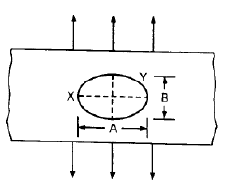Solution:
QUESTION: 6

Consider the following statements:

1. Endurance strength of a component is not affected by its surface finish and notch sensitivity of the material.

2. For ferrous materials like steel, S-N curve becomes asymptotic at 106 cycles.

Which of the statements given above is/are correct?

Solution:

1 is false: affected

QUESTION: 7

When σ and Young's Modulus of Elasticity E remain constant, the energy absorbing capacity of part subject to dynamic forces, is a function of its

Solution:

Strain energy is given by,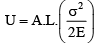∴U is proportional to (A.L.) which is volume.  Also, since U is a function of σ2 , that portion of the part which is prone to high localised will absorb a high amount of energy, making it vulnerable to failure. Such a part, therefore, is designed to have such a contour that, when it is subjected to time-varying or impact loads or others types of dynamic forces, the part absorbs or less uniform stress distribution along the whole length of the part is ensured.

QUESTION: 8

Assertion (A): Soderberg relation is used for design against fatigue.

Reason (R): Soderberg relation is based on yield strength of the material whereas all other failure relations for dynamic loading are based on ultimate strength of the material.

Solution:
QUESTION: 9

In the figure shown, it the line AB represents Goodman criterion of failure, then soderberg criterion could be represented by line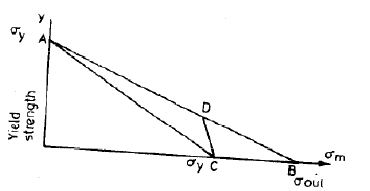Solution:
QUESTION: 10

Assertion (A): The precession of the axis of rotation of a shaft causes a gyroscopic reaction couple to act on the frame to which the bearings are fixed.

Reason (R): The reaction of the shaft on each bearing is equal and opposite to the action of the bearing on the shaft.

Solution:
QUESTION: 11

A bicycle remains stable in running through a bend because of

Solution:
QUESTION: 12

Assertion (A): Endurance limits for all materials are always less than the ultimate strength of the corresponding materials.

Reason (R): Stress concentration in a machine part due to any dislocation is very damaging when the part is subjected to variable loading.

Solution:
QUESTION: 13

Match List I with List II and select the correct answer: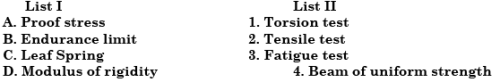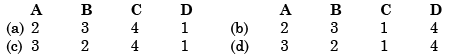Solution:
QUESTION: 14

A band brake having band-width of 80 mm, drum diameter of 250 mm, coefficient of friction of 0.25 and angle of wrap of 270 degrees is required to exert a friction torque of 1000 N m. The maximum tension (in kN) developed in the band is

Solution:
QUESTION: 15

In a band brake the ratio of tight side band tension to the tension on theslack side is 3. If the angle of overlap of band on the drum is 180° the coefficient of friction required between drum and the band is

Solution:
QUESTION: 16

What is the correct sequence of the following steps in engine analysis?

1. Vibration analysis

2. Inertia force analysis.

3.Balancing analysis

4. Velocity and Acceleration analysis.

Select the correct answer using the codes given below:

Solution:
QUESTION: 17

Consider the following design considerations:

1. Tensile failure

2. Creep failure

3. Bearing failure

4.Shearing failure

5. Bending failure

The design of the pin of a rocker arm of an I.C. engine is based on

Solution:

Design of pin of a rocker arm of an I.C. engine is based on bearing, shearing, and bending failures.

QUESTION: 18

Interchangeability can be achieved by

Solution:

Interchangeability can be achieved by standardisation.

QUESTION: 19

Which of the following stresses are associated with the tightening of a nut on a stud?

1. Tensile stresses due to stretching of stud.

2. Bending stresses of stud.

3. Transverse shear stresses across threads.

4. Torsional shear stresses in threads due to frictional resistance.

Select the correct answer using the codes given below:

Solution:
QUESTION: 20

Consider the following statements regarding power:

1. It is the capacity of a machine.

2. The efficiency is always less than unity as every device operates with some loss of energy.

3. A dynamometer can measure the power by absorbing it.

4. Watt-hour is the unit of power.

Of these statements:

Solution: